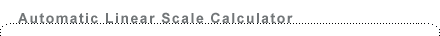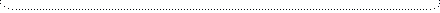Images by Date
Images by Category
Images by Interest
Image Tutorials
Web Shortcuts

### Scale and Distance

 For two points P and Q on the sky at the same distance from Earth, the distance S between these two points can be calculated if the distance D from Earth and the angular distance on the sky A are known. For angular distances less than 10 degrees, the following formula is good to within one percent: S = .0175 D x A(degrees)   (1) IMPORTANT NOTES ON THE LIMITATIONS OF THIS FORMULA: This formula applies if the two points are at approximately the same distance from Earth, for example points on the two edges of the moon, or a star cluster, or a galaxy, or two stars at the same distance. The stars in the constellations are in general NOT at the same distance. For distances greater than about 500 million light years, the effects of the expanding universe must be taken into account, so the simple formula (1) does not apply. See http://www.astro.ucla.edu/~wright/cosmo_02.htm for a detailed discussion of these effects. Example 1. The moon is about 0.51 degree across, and is about 384,000 kilometers from Earth. Using Equation (1), we calculate that the diameter of the moon is approximately: S =( 0.0175) x (384,000) x (0.51) = 3430 kilometers. For more distant sources the angular distance is usually measured in arc minutes or arc seconds. One degree contains 60 arc minutes and 3,600 arc seconds, so Equation (1) is modified as follows: For angular distance in arc minutes, S = .00029 D x A(minutes)  (2) For angular distance in arc seconds, S = .0000048 D x A(arc seconds)  (3)Enter the distance in kilometers or light years and the angular size in degrees to compute the linear scale in kilometers or light years. This calculator is good for angles less than 10 degrees, and distances less than 500 million light years. .0175 x D x A(degrees) =SExample 2. If two stars in a star cluster 800 light years away are 1 arc minute apart, then using Equation (2) their separation in light years is calculated to be:
S = (.00029) x (800) x 1 = .23 light yearsEnter the distance in kilometers or light years and the angular size in arc minutes to compute the linear scale in kilometers or light years. This calculator is good for angles less than 10 degrees, and distances less than 500 million light years. .00029 x D x A(arc minutes) = SExample 3. If a bright star cluster in a galaxy 100 million light years away is 20 arc seconds away from the center of that galaxy, then according to Equation (3), the distance of the star cluster from the center of the galaxy is:
S = (.0000048) (100,000,000)x (20) = 9,600 light years.
Note that in the above formulas, S and D must always be in the same units. That is, if S is in kilometers, D is also in kilometers, etc.Enter the distance in kilometers or light years and the angular size in arc seconds to compute the linear scale in kilometers or light years. This calculator is good for angles less than 10 degrees, and distances less than 500 million light years. .0000048 x D x A(arc seconds) = SIn case you wonder where the numbers in the conversion formulas come from:
The natural unit for measuring angles is the radian, which is derived from the formula for the circumference C of a circle: C = 2πR, where R is the radius. If R = 1, then C = 2π, so the radian is defined such that a circle has 2π radians. It also has 360 degrees, so:
1 radian = 360/2π = 360/6.28 = 57.3 degrees
so